Get instant live expert help with Excel or Google Sheets“My Excelchat expert helped me in less than 20 minutes, saving me what would have been 5 hours of work!”

#### Post your problem and you’ll get expert help in seconds.

Your message must be at least 40 characters
Our professional experts are available now. Your privacy is guaranteed.

# Learn How to Use COUNTIF in Excel

Excel COUNTIF function counts the number of cells in a range that meet specified condition in a range. It is combination of IF and COUNT functions. IF part specifies condition or criteria to check each cell in range and COUNT gives total of those cells which meet that criteria or condition.The syntax for the COUNTIF function is thus combination of two arguments:

`=COUNTIF(range, criteria)`

Range – It consists of one or many cells that contain values to be counted.

Criteria– It contains condition against which each value is tested and counted that meets this condition. Criteria part is not case sensitive. It can be any of the followings;

• A number, such as 60
• Text value or string, such as “West”
• Cell reference,  cell in worksheet containing a value, such as D2
• Expression, a combination of comparison operator and a value, such as “>=60”“<60”“ <>60”.

IF data range contains numbers, then following rules must be followed in criteria part.

• For exact match “=” sign is not used along with number in criteria, such as 10
• Comparison operator, such as “>”“>=””<”“<>” are used along with criterion number in expression. Expression must be enclosed in double quotation marks, such as “>=60”
• In express, comparison operator and cell reference must be joined together using ampersand (&), which is a concatenating operator in Excel. Comparison operator must be enclosed in double quotation marks, but cell reference must not be enclosed in double quotation marks, such as “>=”&D2

IF data range contains text values, then following rules must be followed in criteria part.

• Text value or string must be enclosed in double quotation marks, such as “West”.
• Cell reference containing text value must not be enclosed in double quotation marks
• “?” and “*” wildcard characters can be used for partial match of one and multiple contiguous characters, such as “?ed” or “*ed”

### COUNTIF functions Examples in Excel

In this article we will discuss examples in multiple scenarios. COUNTIF function in Excel is designed to check one criteria or condition on range of values. It is very easy to use COUNTIF function in Excel by following its syntax and rules for numbers and text values as discussed above.

### COUNTIF function for Exact match of Text value or Number

With COUNTIF function in Excel you can easily count the number of values, whether text or number, that are found exactly equal to a specified value in criteria.

As explained earlier, you don’t need to put “=” sign for exact match in criteria part. The only difference between text and numeric value is that text value is placed in double quotation marks and numeric value is specified without double quotation marks in criteria part. But if you use cell reference, that contains either text or number, as criteria then it must be without double quotation marks, like followings;

`=COUNTIF(A2:A10,50)` ➔ Numeric value for exact match criteria without double quotes

`=COUNTIF(B2:B10,”Red”)` ➔ Text for exact match criteria, enclosed in double quotes

`=COUNTIF(B2:B10,C2)` ➔ Cell reference for Text or number exact match without double quotes

Suppose we have score card of top scorer students in a series of tests and we want to count how many times students have scored exactly 90 marks to become top scorer in their tests using COUNTIF function in Excel, such as

`=COUNTIF(D2:D10,90)`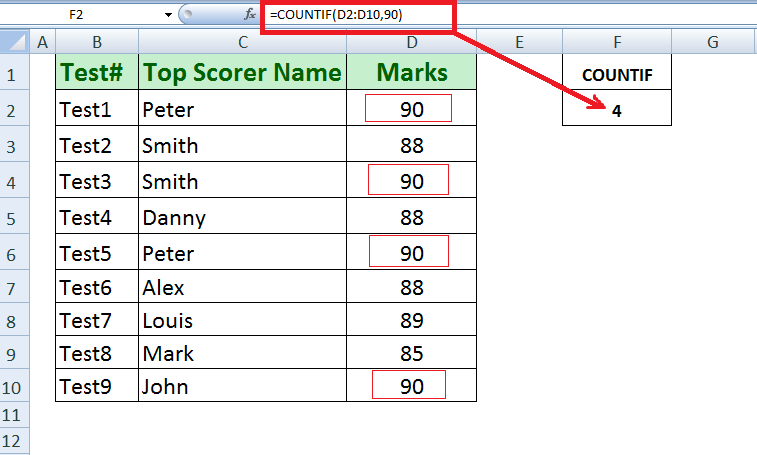Now, using COUNTIF function we can count how many times a certain Student, such as Smith has topped in tests.

`=COUNTIF(C2:C10,"Smith")`

You can see we have changed the range of cells where students’ names are present in table, and specified the criteria value in double quotes.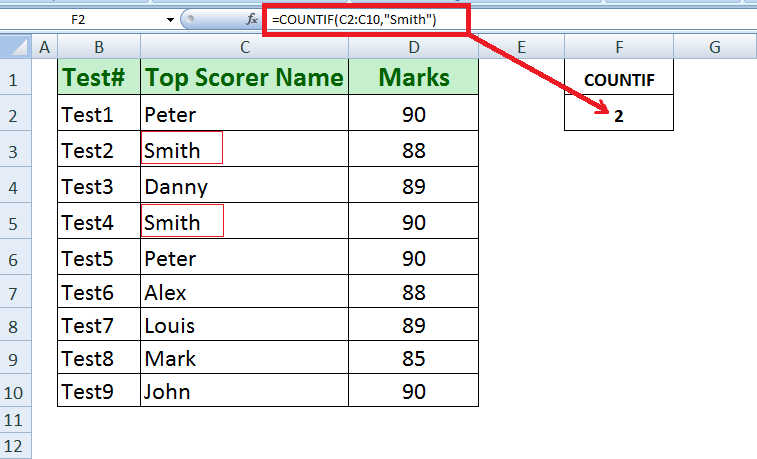You can insert a value in a cell as criteria, whether text or number, and use that cell reference as criteria in COUNTIF function, such as F2

`=COUNTIF(C2:C10,F2)`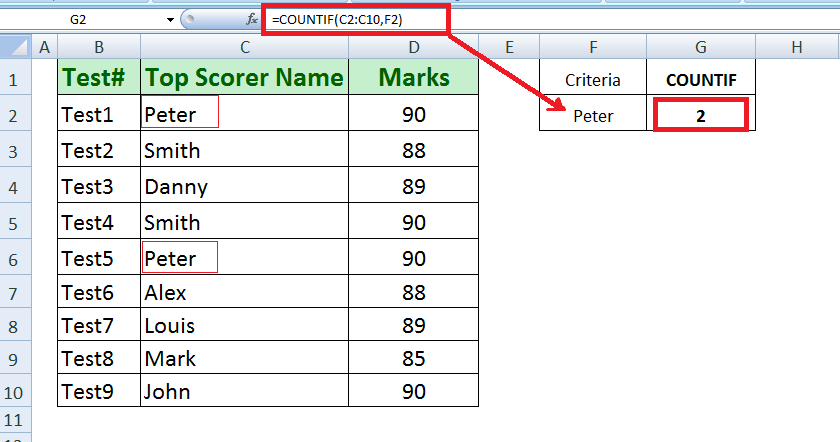### COUNTIF function for Numbers Greater Than, Less Than, Not equal to

As we know criteria can be an expression, containing comparison operator, such as Greater Than (>)Less Than (<)Not equal to (<>) and a number to test the number values against a criteria. Using COUNTIF we can count number of values that meet the condition specified in an expression, such as “>=80”.

Suppose we want to count how many times students have scored Greater Than or equal to 90 marks to top their tests. Using COUNTIF function we can do that easily as given below.

`=COUNTIF(D2:D10,">=90")`

Here, expression “>=90” will test the values in range D2:D10 and COUNTIF function will count the cells that meet this condition.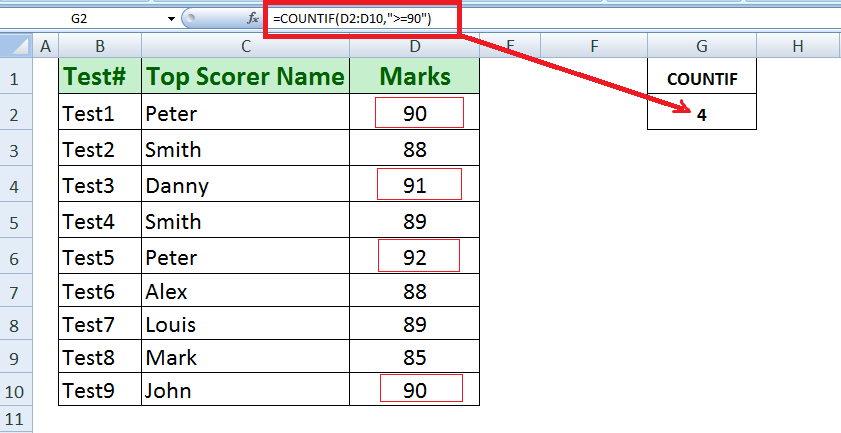We can use cell reference and comparison operator to test this condition in expression, but we need to join comparison operator and cell reference using ampersand (&), such as

`=COUNTIF(D2:D10,">="&F2)`

Here, we need to enclosed comparison operator >= in double quotation marks, but cell reference must be without double quotes, joined by ampersand (&), a concatenating operator.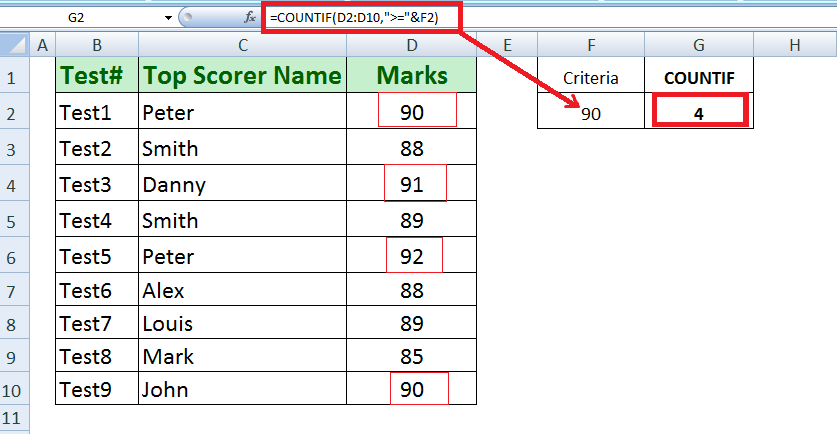### COUNTIF function with wildcard characters

Using COUNTIF function in Excel you can count the text value with partial match using wildcard characters like “?” and “*” for one or multiple contiguous characters.

Suppose we have data of fruit sales of a shop, and we want to count how many varieties of Apple have been sold during a certain period. Using COUNTIF with wildcard “*” containing fruit name we can count the varieties of Apple sold, like;

`=COUNTIF(B3:B13,"*Apple*")`

Using wild card “*” in start and at the end of a text value in criteria part, we can search the specific text value in each and every text string, and COUNT function will count the number of times that text value is found in data range.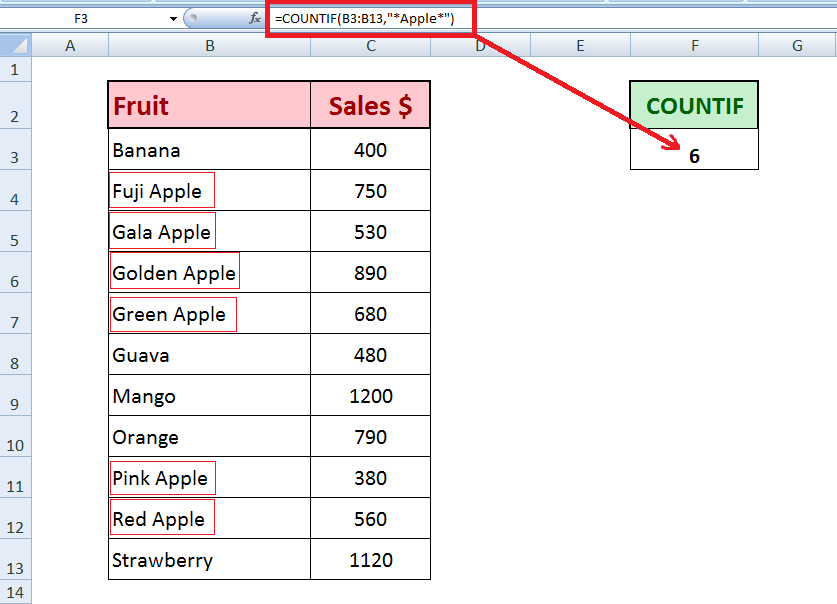If we want to count specific variety of all fruits, even then COUNTIF function can count that for us using “?” or “*” wildcard characters for one or multiple contiguous characters, like;

`=COUNTIF(B3:B14,"*R?d*")`

OR

`=COUNTIF(B3:B14,"*Red*")`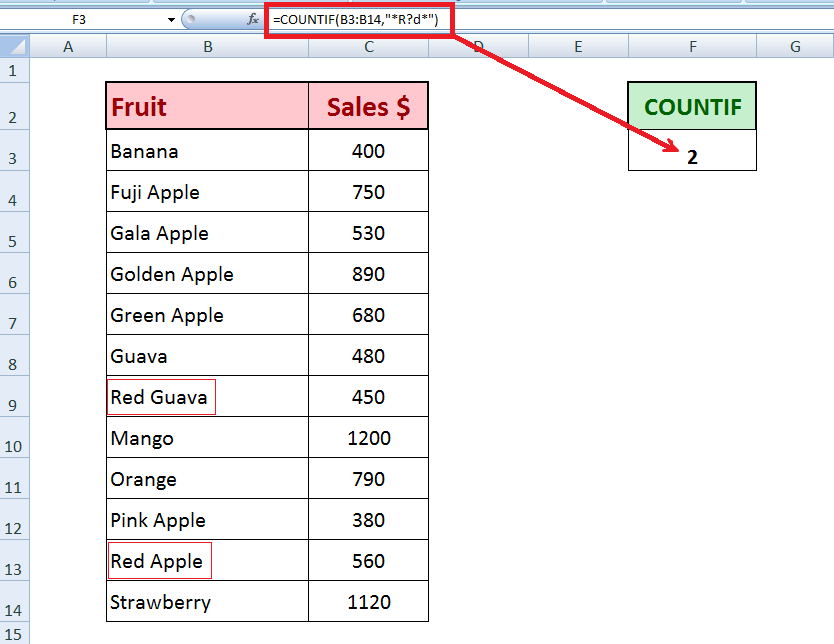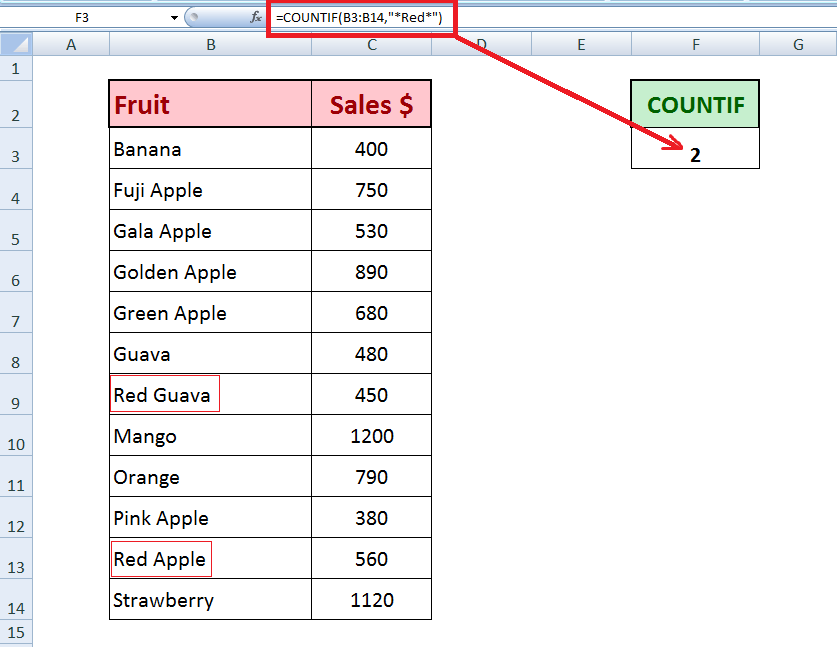### COUNTIF function for blank and Non-blank cells

COUNTIF function we can count number of blank or empty and non-blank cells in a range of cells easily. There are certain methods to do so and we will discuss them one by one.

#### COUNTIF formula for Non-Blank cells

COUNTIF function can count all those cells in a range that contain values. We have a variation in the formula for only text values and all values including text, numeric and date values. We will discuss both methods here.

• COUNTIF for Non-Blank Text values onlyIf we have a range of cells that have numeric and text values, and we want to count those cells that contain only text values, then we use this formula

`=COUNTIF(C3:C14,"*")`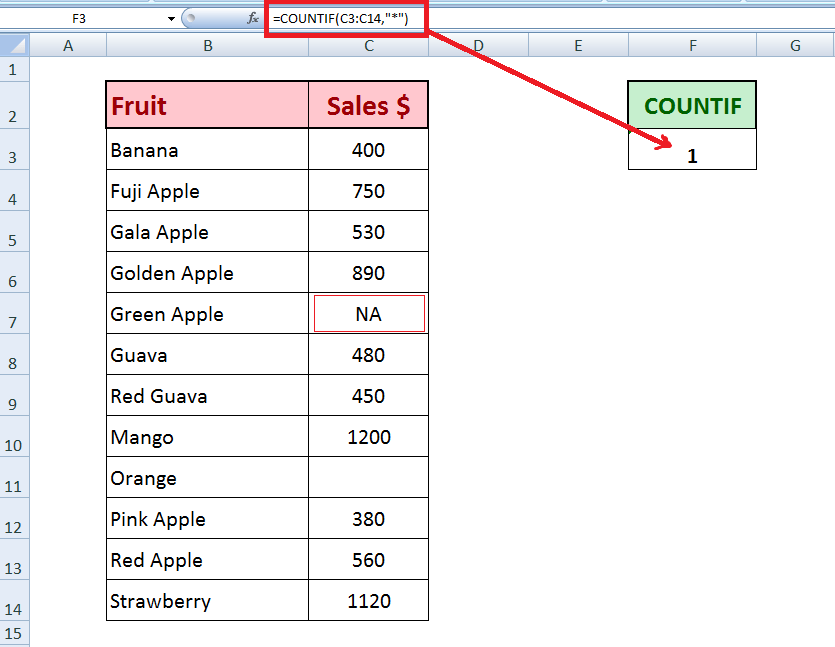• COUNTIF for all Non-Blank valuesIf you want to count all cells that contain values either text, number or dates, then COUNTIF function has little variation in formula. In this formula we will join comparison operator <> (not equal to) and double quotation marks (without space in-between) by ampersand &.

`=COUNTIF(C3:C14,"<>"&"")`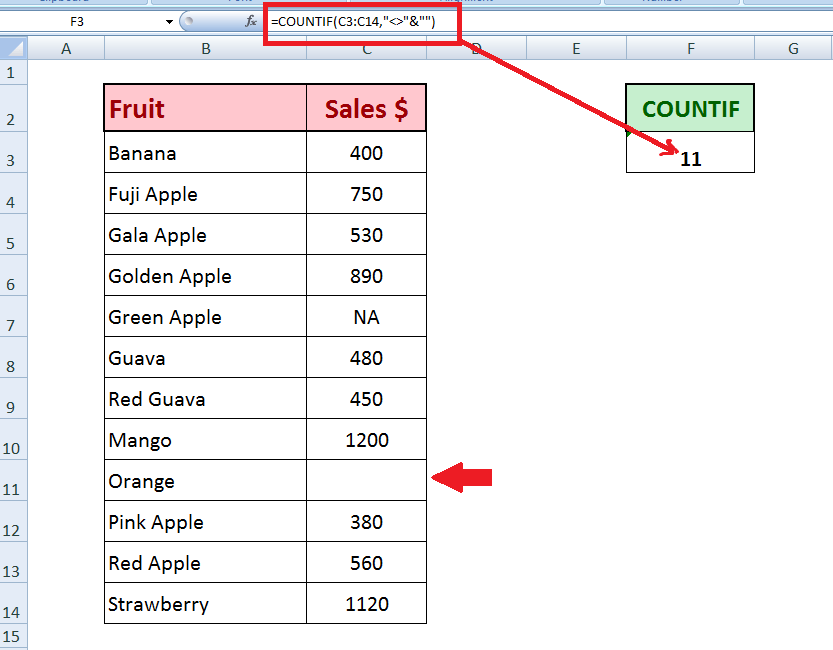#### COUNTIF formula for Blank cells

COUNTIF function can count all those cells in a range that do not contain values. In similar fashion, there is a variation in the formula to count cells that do not contain only text values and those cells that do not contain any type of value, like text, numeric and date values. We will discuss methods here.

• COUNTIF for Non-Text values onlyUsing COUNTIF function we can count all those cells that do not contain Text values in a range, whether they are blank or contain values other than text. This formula uses comparison operator <> (not equal to) and wildcard character “*” joined by ampersand (&).

`=COUNTIF(C3:C14,"<>"&"*”)`

This formula excludes all those cells that contain text values in a range.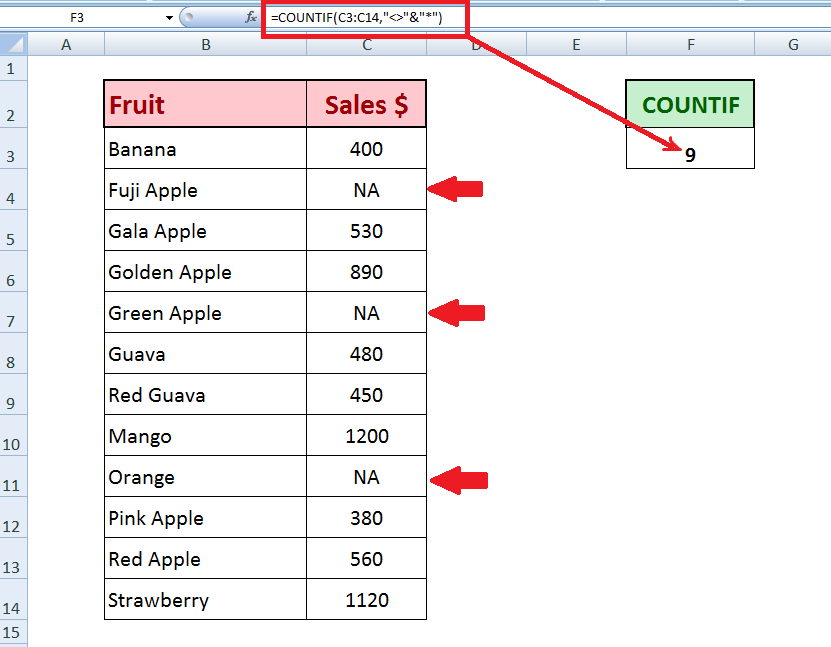• COUNTIF for all Blank cellsIf we want to count cells that are blank and do not contain any type of values including text, numbers, and dates, then we will use following formula using COUNTIF function in Excel.

`=COUNTIF(C3:C14,"")`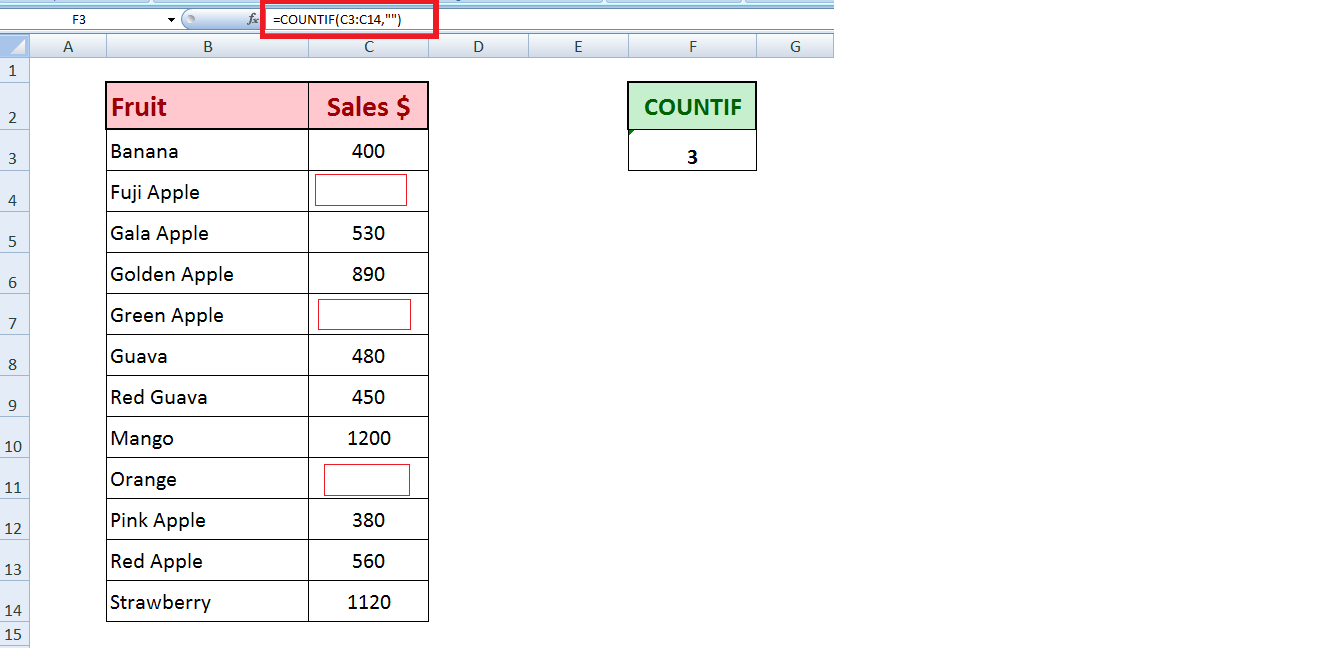#### COUNTIF function for Date values

Dates are specially formatted values and they need extra care while dealing with any function in Excel. In COUNTIF function you also need to follow certain rules while counting the cells that contain date values and also formatted as date, and you put certain criteria to count those cells.

Suppose we have a data set that contains various orders and their expected delivery dates. Using COUNTIF function we can count cells that meet specified condition of dates in criteria. Have a look at following scenarios.

• COUNTIF for exact date valueIf you want to count how many orders are expected to deliver on a certain date, then you must enclose that date in double quotation marks, such as;

`=COUNTIF(D2:D16,"07/02/18")`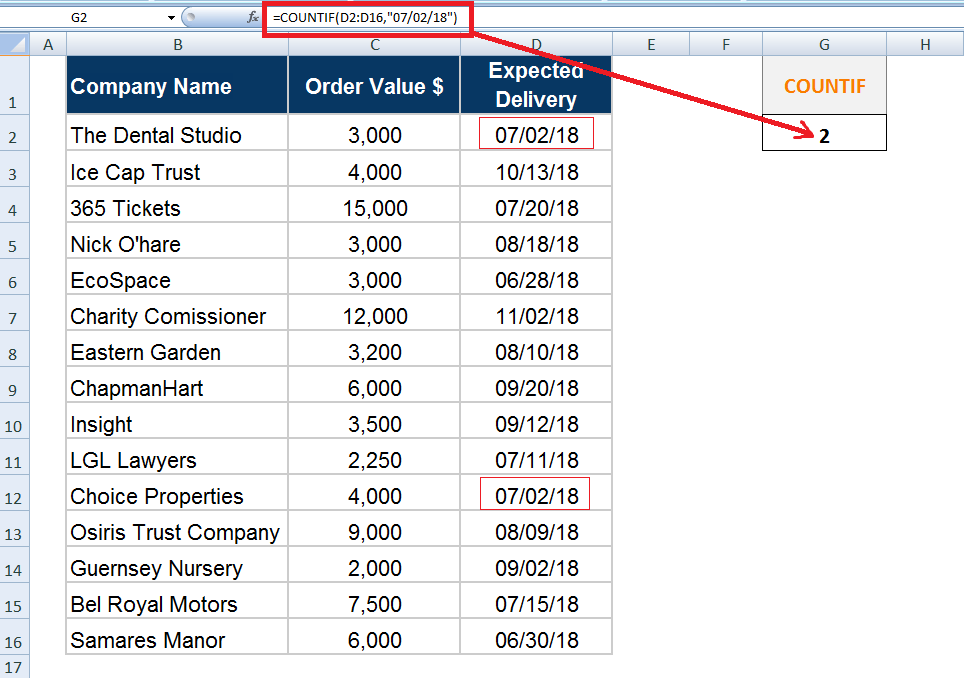But if you use date functions in criteria, like TODAY() function, then you must not enclosed double quotation marks. For example, you want to count how many orders have expected delivery on today’s date, then use following COUNTIF formula;

`=COUNTIF(D2:D16,TODAY())`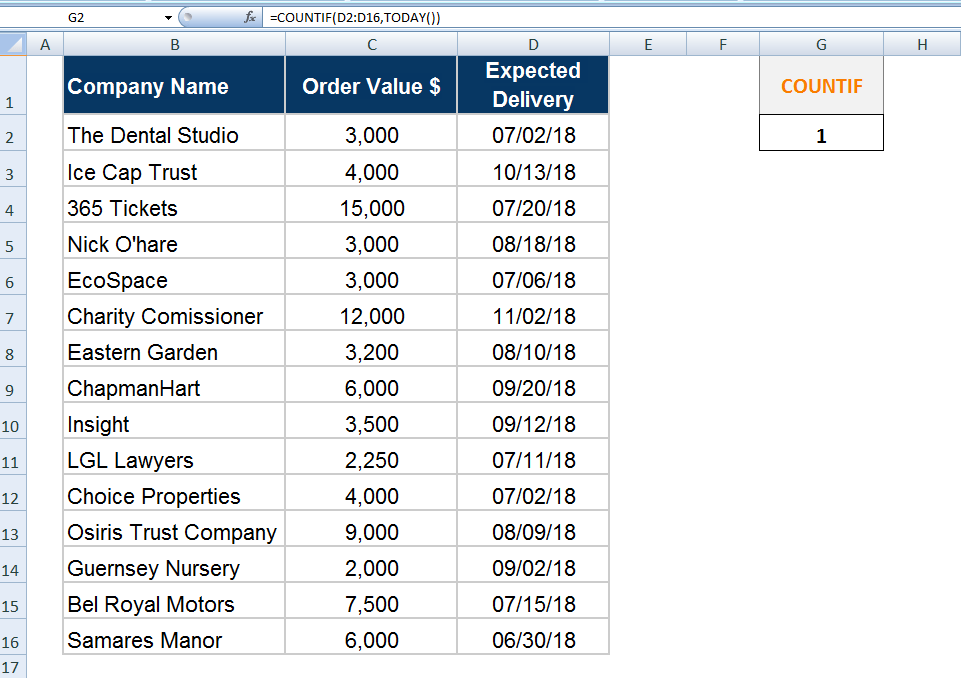• COUNTIF function for Greater Than, Less Than a date valueUsing comparison operator with a date value in expression, we can count how many orders are expected to deliver before a certain future date. Expression must be enclosed in double quotation marks, such as

`=COUNTIF(D2:D16,"<07/20/18")`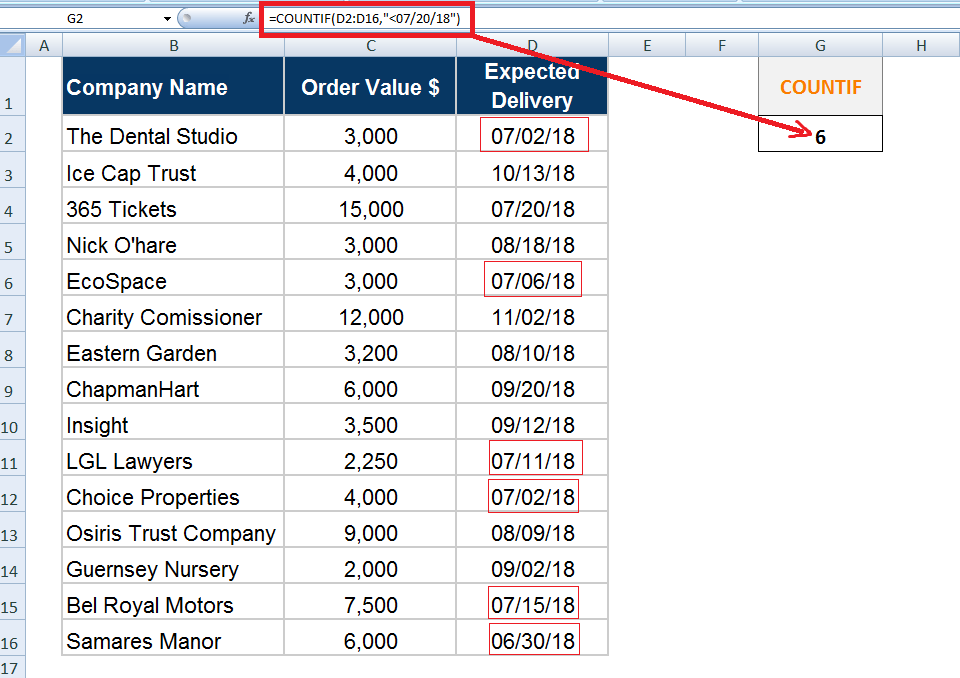We can use cell reference in criteria expression to count how many orders are expected that have expected delivery date greater than equal to a certain date.

`=COUNTIF(D2:D16,">="&F2)`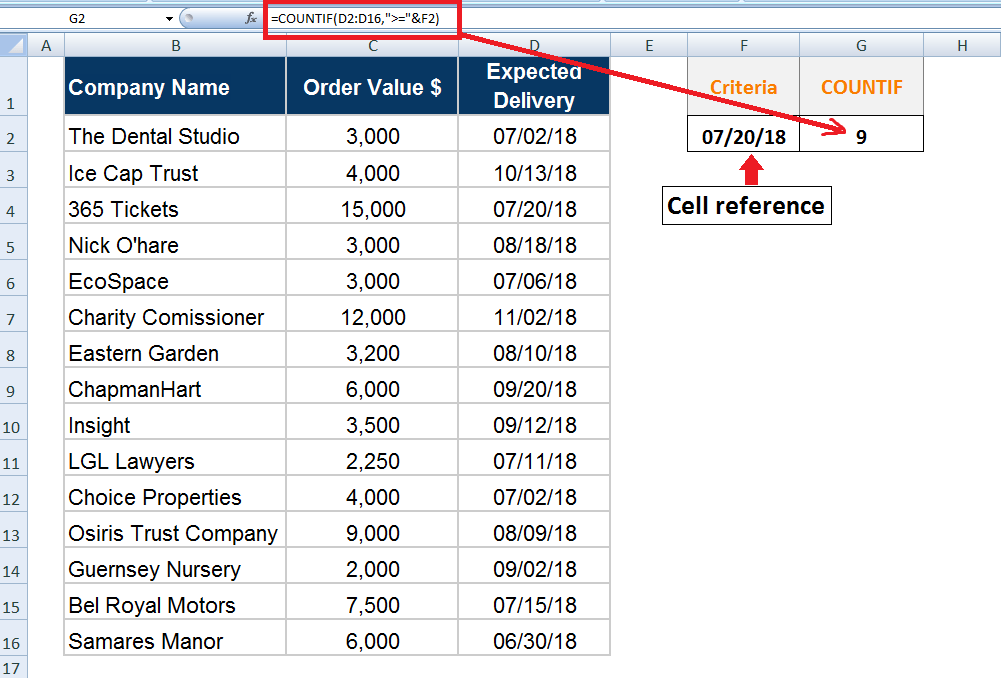COUNTIF Function to find duplicate or unique values

Excel COUNTIF function is used to check occurrence of each value in a range of cells. We can easily find which value has single occurrence (unique value) and which value has multiple occurrences (duplicate value).

Suppose we have a list of City names in a range that have both unique and duplicate values. We can put a logical test in COUNTIF function formula to check whether count of each value is 1 or greater than 1.

In order to do that we will make the range of cells an Absolute range by introducing \$ sign with column and row references in range, such as \$B\$2:\$B\$10. Then we will use cell reference of each value to count its occurrence. Finally we will put a logical test that if count of a value is greater than 1, such as;

`=COUNTIF(\$B\$2:\$B\$10,B2)>1`

We will apply this formula in adjacent cell of 1st value of range and then we will copy it down to all values in range.

IF count of a value is greater than 1, then this formula will return TRUE, representing duplicate occurrence of that value.

IF count of a value is not greater than 1, then it will return FALSE, representing unique occurrence of that value in range.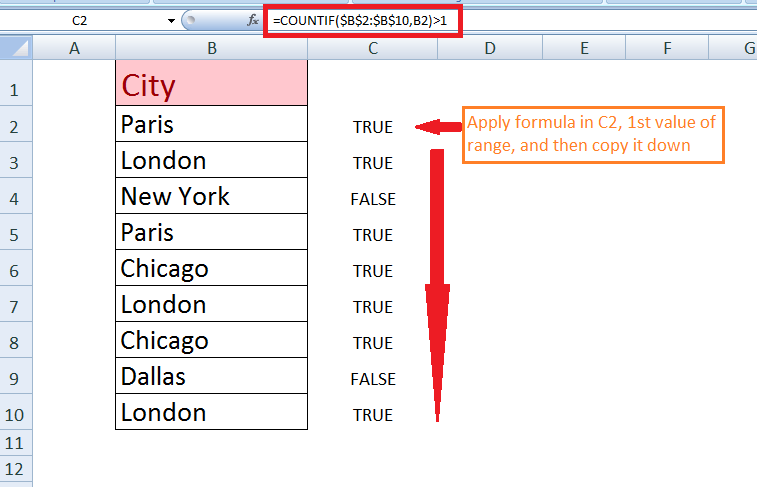Related blogs
Solution examplescan you teach me the steps to create a nested if function, and to nest an AND function inside of an IF function?
Solved by A. Q. in 22 minsI have a list or people who are ranked in numbers from 3 to 6 I need to recognize the contents of each multiple cells and create an equivalent letter. for that value into another column. e.g. Cell E1 = 3 to show in new cell that row (H1) the letter "C" I have multiple rows with different values in column "E" Can this be done?
Solved by I. J. in 30 minsI need a formula to check text values in a range and output one of three possible outputs into a cell. ie: If all text values in cell range = A, then Output 1. If all text values in cell range = B, then Output 2. If there is a mix of A and B values in cell range, then Output 3.
Solved by M. J. in 60 minsCould you help me by explaining the IF function?
Solved by G. L. in 24 minsI want to display a range of cells if they meet a specific criteria. I want to display a name IF it does not equal one of three options AND there are any numbers contained in a range of cells
Solved by G. F. in 60 mins